# A stone

There is a stone weighing 60 kg on the billet. The distance from the support point to the stone is 20cm. The length of the billet is 1m. Determine the force exerted by the hand at the end of the billet.

F2 =  117.72 N

### Step-by-step explanation: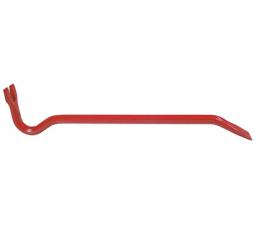Did you find an error or inaccuracy? Feel free to write us. Thank you!Tips to related online calculators
Do you want to convert length units?

## Related math problems and questions:

• Chained winch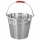When digging a well, a chained winch is used to balance the material. An empty shackle weighs 8 kg, a full 64 kg. What force must the worker exert on the crank handle when pulling the shackle with a uniform movement from a depth of 5 m if the shaft diamet
• Swing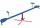A child weighing 12 kg is sitting on a swing at a distance of 130 cm from the rotation axis. How far away from the rotation (center) axis must sit down his mother weighs 57 kg if she wants to be a swing in balance?
• Same force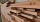The trunk of 5m in length and 95 kilograms has a center of gravity 2m from the thicker end. The tribe is carried by two men, one at the thicker end. At what distance does the trunk carry a man from the other end to make the same force on it?
• Above water surface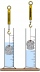If we remove the stone from the water, we apply a force of 120N. How much force will we have to exert if we move the stone above the water surface? The density of the stone is 5000 kg/m ^ 3.
• Two boys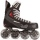Two boys weighing 60kg and 90kg move on roller skates and are attracted to each other by a rope. One boy exerts a force of 15N. What force does the other boy exert? What accelerations will the boys gain? Do not consider friction or air resistance.
• Hydrostatic forceWhat is the hydrostatic force applied to an area of 30 cm2 in the water at a depth of 20 m? (Water density is 1000 kg/m3)
• Positional energyWhat velocity in km/h must a body weighing 60 kg have for its kinetic energy to be the same as its positional energy at the height 50 m?
• Forces on earth directionsA force of 60 N [North] and 80 N [East] is exerted on an object wigth 10 kg. What is the acceleration of the object?
• Brass tubeThe outer perimeter of brass tube (ρ = 8.5 g/cm3) is 38 cm. Its mass is 5 kg, length 54 cm. What is the pipe wall thickness?
• The carThe car weight 1280 kg, increased its speed from 7.3 m/s to 63 km/h on a track of 37.2 m. What force did the car engine have to exert?
• The swing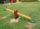To swing the two girls. Aneta weight 45 kg and Simon 35 kg weight. How far should sit Simon from the middle of swing so it is balanced, if we know that Aneta is sitting at distance 1,5m? How far are girls sitting apart?
• Balance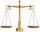The rod are 1.9 m long hanging weights 4 kg and 1 kg on ends. Where are centre of rod (distance from weight 4 kg) to be in balance?
• The bridge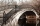A vehicle weighing 5,800 kg passes 41 km/h on an arched bridge with a radius of curvature of 62 m. What force is pushing the car onto the bridge as it passes through the center? What is the maximum speed it can cross over the center of the bridge so that
• Frequency of oscillation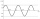A weight of 1 kg was suspended from the spring and the spring was extended by 1.5 cm. Determine the frequency of the natural oscillation of the resulting oscillator.
• Center of gravityThe mass points are distributed in space as follows - specify by coordinates and weight. Find the center of gravity of the mass points system: A1 [1; -20; 3] m1 = 46 kg A2 [-20; 2; 9] m2 = 81 kg A3 [9
• A hammer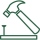A hammer weighing 500 g hits the nail at a speed of 3 m/s. What is the average force acting on the nail, which penetrates the board to a depth of 5 cm?
• Dynamometer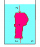What is the volume of a body that stretches a dynamometer in air, on which it is suspended by a force of 2.5 N, and if it is immersed in alcohol with a density of 800 kg/m ^ 3, does it tension the dynamometer with a force of 1.3 N?